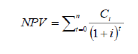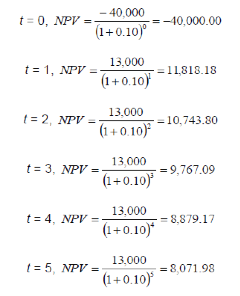## Net Present Value (NPV)

The idea behind the net present value (NPV) is that EUR 1 today is worth more than EUR 1 in the future, because money available today can be invested and grown. NPV is a calculation technique used to estimate the value or net benefit over the lifetime of a particular project, often for long-term investments, such as a dam or a mining project. It allows the decision-maker to compare different alternatives on a similar time scale by converting all options to current monetary figures. A project is considered acceptable (or unacceptable) if the NPV is positive (or negative) over the expected lifetime of the project.

The formula for NPV requires anticipating the period for which (expressed as t, usually in years) money will be invested in the project, the total length of time of the project, the same unit as t), the interest rate (i) and the cash flow at that specific point of time (C, cash inflow or outflow). With these elements, NPV can be calculated as follows:An illustration can be made by way of a business that is considering changing its lighting by switching from incandescent to fluorescent bulbs. The initial investment to change the lights themselves amounts to EUR 40,000. After the initial investment, this project expects to spend EUR 2,000 to operate the lighting system but, on the other hand, it will also yield EUR 15,000 in savings each year. Therefore, there is an annual flow of EUR 13,000 following the initial investment. If the discount rate (or interest rate) is assumed to be 10 percent and the lighting system is utilised over a 5-year period, the project would have the following NPV calculation:This information allows us to calculate the NPV over the lifetime of the project, that is the sum of the 6 rows (from t  = 0 till t  = 5) equals EUR 9,280.22. Notice how much the calculation depends on the interest rate or discount rate. A lower rate will favour the change in lights.

The question arises, why is the discount or interest rate 10 percent, or 5 percent or 2 percent? Does money ‘reproduce’ itself at 10 percent per year? Which are the investments that will sustainably yield such a rate of return, once we take out the value of resource depletion and environmental pollution?

There are several disadvantages to using NPV as an investment criterion, the greatest being sensitivity to the discount rate, as a small increase or decrease will have a considerable effect on the final output. In our example, if we set the discount rate at 15 percent, the NPV equals EUR3,578.02, but a discount rate of 20 percent entails an NPV of EUR -1,122.04. We thus come from a project that creates EUR 9,280.22 of value to one that destroys EUR 1,122.04 instead. The main difficulty then in computing the NPV of a project is determining which discount rate should be used, and how to project future changes in the discount rate.